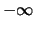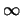BOUNDS data lines are used to define variable bounds that differ from the defaults assumed by the QSopt parser, see Default variable bounds.

Field 1 of a BOUNDS data line contains a bounds indicator, field 2 contains a label, and field 3 a variable name. Depending on the bounds indicator, field 4 is either empty or it contains a floating point number.

Similar to RHS and RANGES data lines, a BOUNDS line is ignored if its label differs from the label used in the first BOUNDS line.

The following table explains the effects of BOUNDS data lines on a variable's bounds. x refers to the variable name and value refers to the number given on the data line.

 indicator uses field 4 interpretation LO, LI yes x ≥ value UP, UI yes x ≤ value FX yes x = value BV no x = 0 or x = 1 MI no x ≥PL no x ≤FR no≤ x ≤The "LI", "UI", or "BV" bounds indicators declare their variable to be an integer variable as well as defining its bounds.